# Visual labeling comparison#

A first important step when comparing segmentation algorithms is to visualize the results of the individual algorithms and showing differences between them.

import numpy as np
from pyclesperanto_prototype import imshow
import matplotlib.pyplot as plt
import pandas as pd


From just visualizing the images, we can see that none pair of the four compared algorithms produce the same label image.

filenames = [
"blobs_labels_imagej.tif",
"blobs_labels_skimage.tif",
"blobs_labels_clesperanto_python.tif",
"blobs_labels_clesperanto_imagej.tif"
]

for filename in filenames:
print(filename)
imshow(label_image, labels=True)

blobs_labels_imagej.tif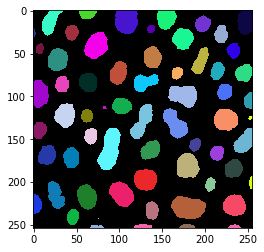blobs_labels_skimage.tif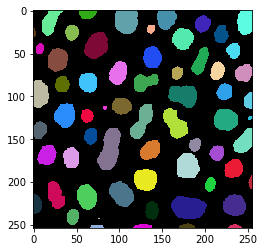blobs_labels_clesperanto_python.tif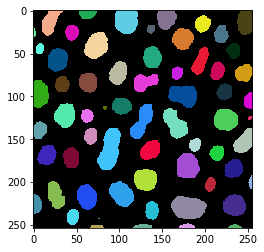blobs_labels_clesperanto_imagej.tifAs corresponding objects have different colours in this visualization, we can conclude that at least the labels of the objects are differnt. We cannot conclude yet if Otsu’s thresholding method delivers different results in the given implementations.

## Difference images#

To see the overlap between the different images, we will produce binary images from the label images and visualize the difference between these binary images.

We first compare ImageJ’s result and scikit-image’s result to demonstrate the procedure.

binary_blobs_imagej = imread(filenames) > 0
imshow(binary_blobs_imagej)
imshow(binary_blobs_skimage)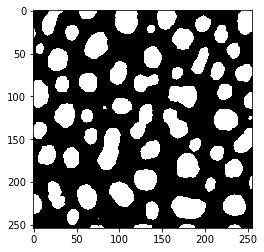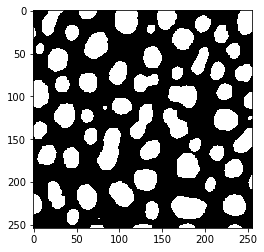When looking at these two images carefully, one can already see that objects are a tiny bit larger in the second image.

To produce the difference image, we will use numpy’s logical_xor method. It will set all pixels in the difference image to 1, where either of the two input binary images are 1, but not both. It is called the exclusive-OR operator.

difference = np.logical_xor(binary_blobs_imagej, binary_blobs_skimage)

fig, axs = plt.subplots(figsize=(10,10))
axs.imshow(difference)

<matplotlib.image.AxesImage at 0x24619eb5130>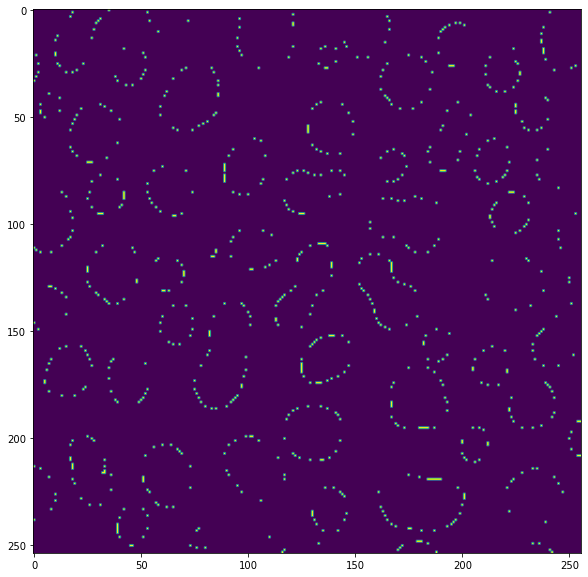From this binary image, we can count the number of pixels that are different between these two approaches:

np.sum(difference)

830


## A matrix of difference-pixel counts#

We will now program two for-loops that iterate over pairs of label images and compare them using the same approach. The result will be a 4x4 matrix with the number of pixels that are different when comparing the corresponding algorithm implementations.

matrix = {"Algorithms": filenames}

for filename_A in filenames:
measurements = []
for filename_B in filenames:

difference = np.logical_xor(image_A, image_B)
number_of_different_pixels = np.sum(difference)
measurements.append(number_of_different_pixels)

matrix[filename_A] = measurements

pd.DataFrame(matrix)

Algorithms blobs_labels_imagej.tif blobs_labels_skimage.tif blobs_labels_clesperanto_python.tif blobs_labels_clesperanto_imagej.tif
0 blobs_labels_imagej.tif 0 830 830 830
1 blobs_labels_skimage.tif 830 0 0 0
2 blobs_labels_clesperanto_python.tif 830 0 0 0
3 blobs_labels_clesperanto_imagej.tif 830 0 0 0

From this matrix, we can conclude that only the ImageJ result is different from the other three implementations.

## Exercise#

We should also quickly confirm that by looking at an other difference image, for example between the clesperanto-implementation in Jython and scikit-image in Python. Visualize both binary images and the difference image between these two. Also count the number of pixels that are different between these two images.